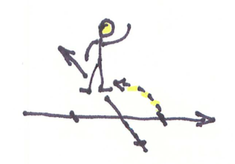Kinesthetics

# Kinesthetic Intro to Complex NumbersHenri Picciotto

This is based on a presentation by Michael Pershan and Max Ray. For their whole unit, go to Michael's site. Their approach assumes some exposure to the basic geometric transformations.
Here is a version of what they presented, as filtered by my memory and beliefs.

Mark some spots on a number line on the ground, representing the integers from (say) -10 to 10. Have a student stand on each integer from -5 to 5. Tell them you'll be calling out instructions using basic arithmetic, which they must follow accurately and gracefully. If students ask why it needs to be graceful, tell them that one should seek beauty always.

Call out "Add 1" Students should move to the next spot on the number line. Ask: "What transformation was that?" (A translation, vector +1) After every move, call "Go back!" to get them to return to the starting position. Repeat with the following moves:

• "Subtract 2" (translation) "Go back!"
• "Add 4" (translation) "Go back!"

Now ask: "How could I have called the last move with two consecutive identical moves?" (Translation with vector +2, twice.)

At this point, insert a brief discussion: "How could I do a translation by +100 in two consecutive identical moves? (+50) How about a translation by x? (\frac 1 2x)"

Repeat the whole routine with multiplication, which will yield a dilation centered at the origin. This time, instead of moving in unison, the students will spread out (or in). Remind the students to do this accurately and gracefully.

• "Times \frac 1 2" (dilation) "Go back!"
• "Times 2" (dilation) "Go back!"

For the next step, limit yourself to three students, starting on -1, 0, and 1. "Times 9" (dilation) "Go back!" "Why are we just doing this with three students?" (Alternate approach: do it with everyone at first, and it will make clear why limit it to three students.) "How could I do this last move in two consecutive identical moves?" (Two dilations with scaling factor 3.) "How could I do a dilation with scaling factor 100 in two identical moves? (times 10.) How about scaling factor x?" And voilà, square root has come into the picture.

Once again, with students standing from -5 to 5, call "Times -1" Chaos will likely ensue as students find their way to the other side. Insist that they start over, and this time do it in some sort of coordinated, graceful way. If they can't seem to find a way to do that, ask: "What sorts of transformations do you know about? Perhaps one of those would help?" Hopefully that will inspire them to make this move as a 180° rotation, which is quite manageable as an accurate and graceful move.

"How could we do this in two consecutive identical moves?" (two 90° rotations) Starting from the home position, ask them to do the first of the two moves, in the positive direction. Holding hands may help keep the distances between them constant. They should end up on a line perpendicular to the original number line, through zero. "Where are you standing?" (On the y-axis.) "What did we multiply by?" (the square root of -1).

At which point you can go back to the classroom, and debrief. Announce that while all the numbers they had seen until today can be found on the number line, they have just encountered a number, i, that is off the number line.

Tell them that all points on the plane can represent numbers, and those are called complex numbers. Introduce a+bi notation, with the rule that i^2=-1. Practice multiplying by i with this rule. For example, start with 2+3i, multiply it by i, then multiply the result by i, and so on. Students will see that multiplying by i amounts to a 90° rotation.

Pershan and Ray generalize to other angles by using fractional powers of -1, but I would suggest that this would be a good time to introduce polar form. The introductory work up to this point is sufficient introduction to the idea that when multiplying complex numbers in polar form, we add the angles.